# ISREF Function

Checks if a given value is a reference or not

## What is the ISREF Function?

The ISREF Function is categorized under Excel Information functions. The function will test if a given value is a reference or not. If the given value is a reference, it will return TRUE. Otherwise, it will return FALSE.

In financial analysis, let’s assume we need to create 21 worksheets in a workbook. All 21 worksheets must be assigned a name. Hence, to avoid missing a sheet, we can use ISREF and keep track of them.

### Formula

=ISREF(values)

The ISREF function uses only one argument:

1. Values (required argument) – This is the given value or expression that we wish to test.

### How to use the ISREF Function in Excel?

As a worksheet function, ISREF can be entered as part of a formula in a cell of a worksheet.

#### Example 1

Let’s see a few examples to understand how this function works: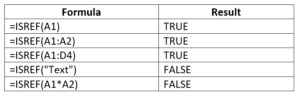We get the results below: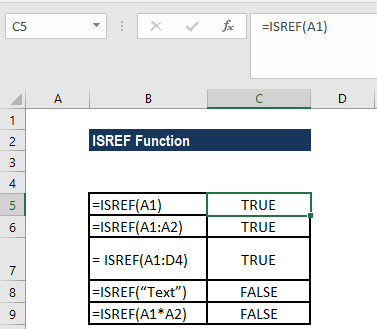In cell B9, we provided expression A1*A2, which would return a value that is a product of the values in two cells. Hence, in this case, the ISREF function will return FALSE.

#### Example 2

Let’s now see how to test if a worksheet name exists in the workbook. To do that, we can use a formula that is based on the ISREF and INDIRECT functions. Suppose we want to find out if the following worksheets exist or not: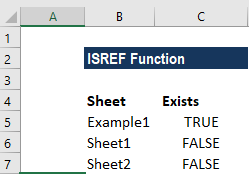The formula used is =ISREF(INDIRECT(“sheetname”&”!A1″)), as shown below: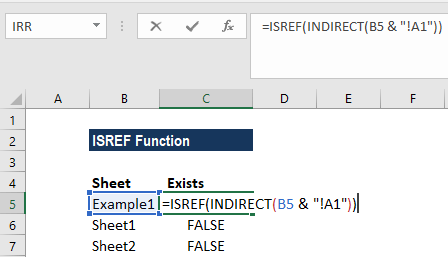We get the following results: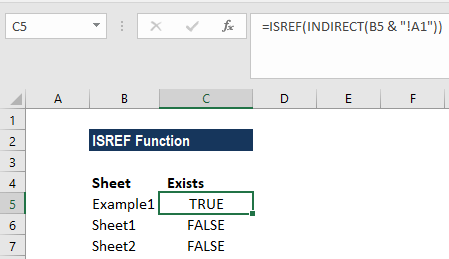The ISREF function used here will return TRUE for a valid worksheet reference and FALSE if the reference is invalid.

In this case, as we want to find out if a particular sheet exists in a workbook or not, we construct a full reference by concatenating the sheet names in column B with an exclamation mark and “A1”:

B5 &”!A1”

The formula returns the text: “Example1!A1!” The text goes into the INDIRECT function. INDIRECT then tries to evaluate the text as a reference.

When INDIRECT succeeds, the reference is passed into ISREF, which returns TRUE. When INDIRECT can’t create a reference, it throws a #REF error, and ISREF returns FALSE.

Thanks for reading CFI’s guide to important Excel functions! By taking the time to learn and master these functions, you’ll significantly speed up your financial analysis. To learn more, check out these additional CFI resources:

• Excel Functions for Finance